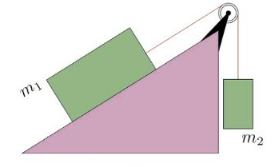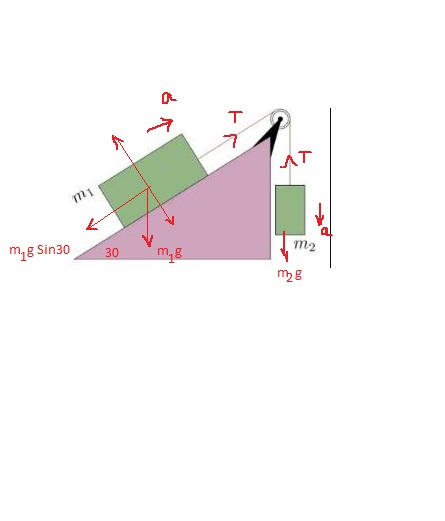# A block with mass m1 = 4:50 kg on a frictionless incline ( = 30) is connected by a cord over a...

## Question:

A block with mass m1 = 4:50 kg on a frictionless incline ( = 30) is connected by a cord over a massless, frictionless pulley to a second block of mass m2 = 3:00 kg, as shown in the Figure 6 below.a)Find the magnitude of the acceleration of block 1

b)Find the magnitude of the acceleration of block 2

c)What is the direction of the acceleration of block 2?

d)What is the tension in the cord?

e)For what value of m2/m1 will the blocks have no acceleration?

## Newton's Second Law

According to Newton's second law, the net force on an object is given by the product of mass and acceleration of the object. If the object is at rest then force on it would be zero.

## Answer and Explanation:(a)

Let us consider the acceleration of the system is "a"

Considering the FBD of mass 2

{eq}\displaystyle m_{2}g - T = m_{2}a \\ (3*9.81) - T = 3a \\ T + 3a = 29.43 \ -------------(1) {/eq}

Now considering the FBD of mass 1

{eq}\displaystyle T - m_{1}g \sin30 = m_{1}a \\ T - (4.5*9.81\sin30) = 4.5a \\ T - 4.5a = 22.07 \ ----------(2) {/eq}

On solving 1 and 2 , we get

{eq}\displaystyle a = 0.981 \ m/s^{2} \\ T = 26.49 \ N {/eq}

Therefore the accleration of the block 1 would be 0.981 m/s^{2}

(b)

Acceleration of both the block would be same, therefore acceleration of the block 2 would be {eq}\displaystyle 0.281 \ m/s^{2} {/eq}

(c)

Since the acceleration has come to positive, therefore our assumed direction is correct.

Therefore the direction of acceleration will be downward for the mass 2.

(d)

We have already found that the tension in the cord is 26.49 N

(e)

If there is no acceleration, then equation 1 would be

{eq}\displaystyle T = m_{2}g {/eq}

Now, for the mass 1

{eq}\displaystyle T= m_{1}g \sin30 {/eq}

On taking the ratio of the above equations

{eq}\displaystyle \dfrac{m_{2}g}{m_{1}g \sin30} = 1 \\ \dfrac{m_{2}}{m_{1}} = \sin30 \\ \dfrac{m_{2}}{m_{1}} = \dfrac{1}{2} {/eq}

#### Learn more about this topic:Newton's Laws of Force & Motion

from GED Science: Life, Physical and Chemical

Chapter 7 / Lesson 6
9.6K